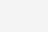# 启发式参数优化算法举例

## 背景## 实例

$$f(x)=22.71282-20*e^{-0.2*\sqrt{0.5*(x_{1}^{2}+x_{2}^{2})}}-e^{0.5*(2*\pi*(x_1+x_2))}$$## 遗传算法### 初始种群

$$InitPopulation=function(npoint,ndim,factor[ndim,2]){…return\quad Population_0}$$

### 进化

#### 选择

$$SelectSpecies=function(Populationbeforeselect_{i}){…return \quad Populationafterselect_{i}}$$
$$Populationbeforeselect_{i}$$表示第i代还没有经过选择的种群，相应的输出则为$$Populationafterselect_{i}$$则表示本次输出第i代选择后的种群。

#### 遗传

$$Hereditary = function(Population_{i},Gprob){…return \quad Population_{i+1}}$$其中$$Population_{i}$$是遗传输入的第i代种群，输出$$Population_{i+1}$$是遗传后产生的第i+1代子代，在这部中存在一个遗传率Gprob，它表示对每一个个体有Gprob的可能性需要通过遗传将个体的基因信息传递给子代。因此这一步的关键是需要选择父体和母体，然后交换两者一半的基因值（二分交换或者奇偶交换可以自由选择）。在种群中循环执行以上步骤，即可完成第i代种群向i+1代进化的目的。

#### 变异

$$MutationGene=function(Populationbeforemutation_{i+1},Cprob，ndim,factor[ndim,2]){…return \quad Populationaftermutation_{i+1}}$$,其中$$Populationbeforemutation_{i+1}$$是遗传过程得到的第i+1代种群，$$Populationaftermutation_{i+1}$$则是第i+1代种群实现部分变异的种群，在这其中有一个关键的参数就是Cprob（变异率），表示种群中的个体发生变异的可能性。这个函数的处理过程就借助突变率来选择可能发生突变的个体，并借助ndim和factor[ndim,2]这两个基因条件生成新的基因组合来更新第i+1代种群中个体的基因值。最终输出第i+1代变异后种群。

## 粒子群算法

### 粒子群更新

$$v(x)=w*v+c_1*r_1*(pbest-x)+c_2*r_2*(gbest-x)$$

$$x_{i+1}=x_i+v(x_i)$$## 实验结果

$$参数：c_1 = 0.1，c_2 = 1，w = 0.1，npoint=50$$
$$结果：result =[ 0.0004,-0.01153]–>f（result）= 1.098832$$

$$参数：npoint=50；Gprob=0.85，Cprob=0.15$$
$$结果：result = [0.04385699 -0.01490619]–>f(result) = 1.208231$$## 总结

1. 仿生算法
2. 随机初始化种群
3. 根据最优值进化

1. 使用场景：粒子群算法适用于连续参数优化，遗传算法适用于离散参数优化
2. 实现：粒子群算法没有遗传、变异过程，实现比较简单，而遗传算法相对复杂。